几种排序算法的简单总结 转

离职总是很麻烦，老板人不错，得好好把工作对接完咯。晚上闲一下就写写博客。

前言

下面会讲到一些简单的排序算法（均基于java实现），并给出实现和效率分析。

使用的基类如下：

注意：抽象函数应为public的，我就不改代码了

public abstract class Sortable {
protected String LABLE="排序算法";
//比较两个数（使用了Integer中sort的源码）
protected int compare(int x, int y) {
return (x < y) ? -1 : ((x == y) ? 0 : 1);
}
//同上，不过返回改为bool
protected boolean less(int x,int y){
return compare(x,y) <0;
}
//交换数组中的两个值
protected void exch(int[] a,int i,int j){
Integer temp = a[i];
a[i] = a[j];
a[j] = temp;
}
//子类需要实现的排序算法
public abstract void sort(int[] a);
public String getLABLE() {
return LABLE;
}
}

冒泡排序

最常见的，毕竟老师教给我们的的第一种排序算法。实现起来很简单，不过实际应用很少（正常情况下），复杂度O(n²)。

原理

趟一趟的比，每一趟中，循环剩余的数，和后一个进行比较，若比它小则交换。这样一趟下来最小的在第一个，最大的在最后一个。总共比n-1趟。

实现

import com.anxpp.sort.base.Sortable;
/**
* 最简单的冒泡排序
*
* @author anxpp.com
* 原理：比较相邻两个元素，从第一对开始比较一直到最后一对，若顺序不对就交换（感觉就像冒泡一样）。
*       一趟比较后，最大（或最小）的会位于最后的位置，然后再以类似方式比较前面的元素。
*/
public class BubbleSort extends Sortable {
public BubbleSort(){
super.LABLE = "冒泡排序";
}
@Override
public void sort(int[] a) {
for(int i=0;i<a.length-1;i++){
for(int j=0;j<a.length-1-i;j++){
if(less(a[j+1],a[j])){
exch(a,j,j+1);
}
}
}
}
}

优化

上面的算法，无论的你的数据怎么样，始终都要比n²次，效率很低。若你的数据局部有序，经过几趟交换以后，已经有序，则不用继续往下比。效率会高很多（绝大多数情况下）。优化代码如下：

import com.anxpp.sort.base.Sortable;
/**
* 设置标志优化后的冒泡排序
* @author anxpp.com
*
* 原理：比较相邻两个元素，从第一对开始比较一直到最后一对，若顺序不对就交换（感觉就像冒泡一样）。
*       一趟比较后，最大（或最小）的会位于最后的位置，然后再以类似方式比较前面的元素。
* 优化：传统的冒泡排序，总是要比较那么多次，如果在某趟完成后，并无交换表示数据已经有序，所以设置
*       一个标志，如某趟比较完成后没有发生，则不再继续后面的运算直接返回即可，其实，有时候效率反而会比传统的低！
* 其他：据说分而治之也能有用到冒泡，这里就不深究了...
*/
public class BetterBubbleSort extends Sortable {
public BetterBubbleSort(){
super.LABLE = "冒泡排序优化";
}
@Override
public void sort(int[] a) {
boolean didSwap;
for(int i=0;i<a.length-1;i++){
didSwap = false;
for(int j=0;j<a.length-1-i;j++){
if(less(a[j+1],a[j])){
exch(a,j,j+1);
didSwap = true;
}
}
if(!didSwap) return ;
}
}
}

选择排序

和冒泡复杂度一样O(n²)，但是时间上可能会比冒泡稍微快一点，因为交换的次数比冒泡少。

原理

选择排序可以说是最好理解的算法。就是每次遍历一趟，找出最小的数，放到最前端。（这里说的是最前，是指无序的队列中的最前）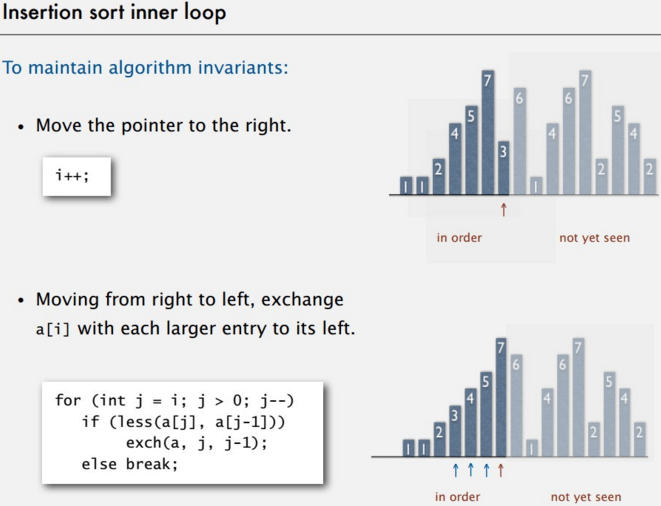实现

import com.anxpp.sort.base.Sortable;
/**
* 选择排序
* @author anxpp.com
*
*/
public class SelectionSort extends Sortable {
public SelectionSort(){
super.LABLE = "选择排序";
}
@Override
public void sort(int[] a) {
for(int i=0;i<a.length;i++){
int min=i;
for(int j=i+1;j<a.length;j++){
if(less(a[j],a[min])){
min = j;
}
}
exch(a,i,min);
}
}
}

插入排序

时间复杂度O(n²)。

原理

遍历未排序序列。把未排序数列的第一个数和已排序数列的每一个数比较，若比它大则交换。经典的理解方式就是理解成摸牌时候理牌的顺序。我上面的实现是直接交互数字，若是把大的数直接往后移效率还会更高。

实现

import com.anxpp.sort.base.Sortable;
/**
* 插入排序
* @author anxpp
*
*/
public class InsertionSort extends Sortable {
public InsertionSort(){
super.LABLE = "插入排序";
}
@Override
public void sort(int[] a) {
for(int i=1;i<a.length;i++){
for(int j=i;j>0;j--){
if(less(a[j],a[j-1])){
exch(a,j,j-1);
}
else break;
}
}
}
}

适合插入排序的数据

当你的数据是基本有序的时候且数据量小，利用插入排序的时候，效率会很高。若数据为逆序的话，效率很低。

希尔排序

可以看出是插入排序的一种优化，或者是预处理。希尔排序就是先进行h-sort，也就是让间隔为h的元素都是有序的。普通的插入排序就是1-sort。

原理

主要就是选定一个h的有序数组来进行预排序。这样最后进行插入排序的时候，能使数据局部有序。就算交换的话，交换的次数也不会很多。这样h序列称为递增序列。希尔的性能很大部分取决于递增序列.一般来说我们使用这个序列3x + 1.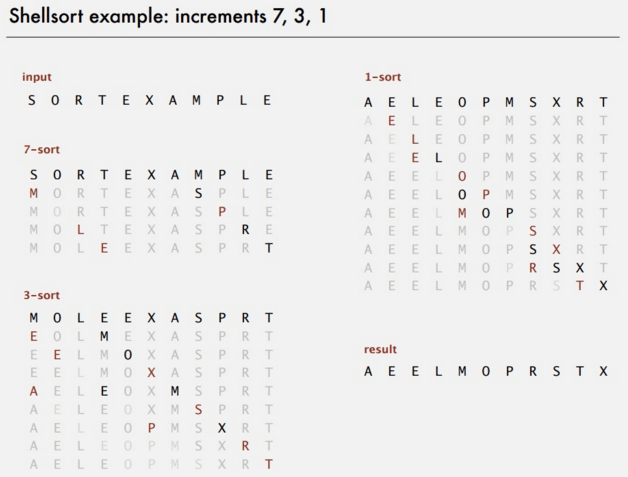实现

import com.anxpp.sort.base.Sortable;
/**
* 希尔排序
* @author anxpp.com
*
*/
public class ShellSort extends Sortable {
public ShellSort(){
super.LABLE = "希尔排序";
}
@Override
public void sort(int[] a) {
int h=1;
while(h<a.length/3){
h=3*h+1;
}
while(h>=1){
for(int i=h;i<a.length;i++){
for(int j=i;j>=h;j=j-h){
if(less(a[j],a[j-h])){
exch(a,j,j-h);
}
else break;
}
}
h=h/3;
}
}
}

性能

对于希尔排序的性能其实无法准确表示。介于O(nlogn)和O(n²)之间，大概在n的1.5次幂左右。

希尔排序对于中大型数据的排序效率是很高的，而且占用空间少，代码量短。而且就算是很大的数据，用类似快排这种高性能的排序方法，也仅仅只比希尔快两倍或者不到。

归并排序

复杂度O(nlogn).

核心思想就是采用分而治之的方法，递归的合并两个有序的数组。效率比较高，缺点是空间复杂度高，会用到额外的数组。

原理

核心代码是合并的函数。合并的前提是保证左右两边的数组分别有序，在合并之前和之后在Java中我们可以用断言来保证数组有序。合并的原理其实也很简单，先把a数组中的内容复制到额外储存的temp数组中去。分别用两个index指向a数组的起始位置和中间位置，保证a数组左右两边有序，比如i，j。现在开始从头扫描比较左右两个数组,若a[i]<=a[j]，则把a[i]放到temp数组中去，且i向前走一步。反正则放a[j]，且j走一步。若其中一个数组走完了，则把另一个数组剩余的数直接放到temp数组中。我们用递归的方式来实现左右两边有序。递归到数组只有1个数时肯定是有序的，再合并2个数，再退出来合并4个数，以此类推。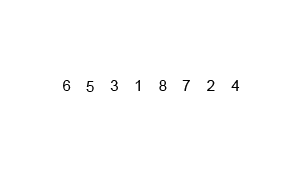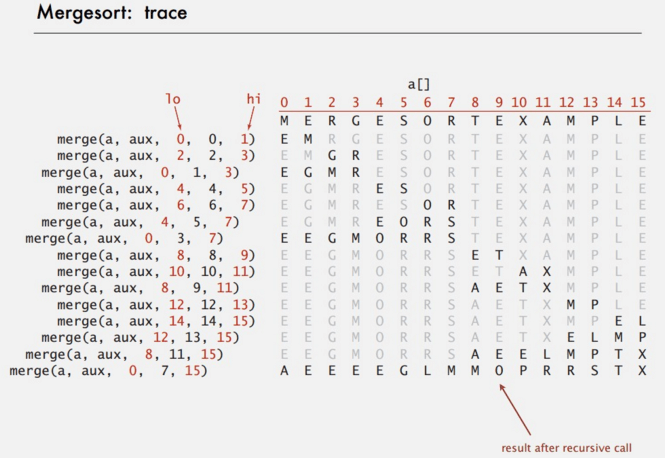实现

import com.anxpp.sort.base.Sortable;
/**
* 归并排序
* @author anxpp.com
*
*/
public class MergeSort extends Sortable {
public MergeSort(){
super.LABLE = "归并排序";
}
int[] temp ;
private void merge(int[] a, int l, int m, int h){
for(int i=l;i<=h;i++){
temp[i]=a[i];
}
int i=l;
int j=m+1;
for(int k=l;k<=h;k++){
if(i>m) a[k]=temp[j++];
else if(j>h) a[k]=temp[i++];
else if(less(temp[i],temp[j])) a[k]=temp[i++];
else a[k] = temp[j++];
}
}
private void sort(int[] a,int l,int h) {
if(l<h){
int mid = (l+h)/2;
sort(a,l,mid);
sort(a,mid+1,h);
if (!less(a[mid+1], a[mid])) return;
merge(a,l,mid,h);
}
}
@Override
public void sort(int[] a) {
temp = new int[a.length];
sort(a,0,a.length-1);
}
}

优化

归并排序对小数组排序时，由于会有多重的递归调用，所以速度没有插入排序快。可以在递归调用到小数组时改采用插入排序。小数组的意思是差不多10个数左右。

如果递归时判断已经有序则不用继续递归。也可以增加效率。

private void sort(int[] a,int l,int h) {
if(l<h){
int mid = (l+h)/2;
sort(a,l,mid);
sort(a,mid+1,h);
if (!less(a[mid+1], a[mid])) return;
merge(a,l,mid,h);
}
}

另外在合并时交互两个数组的顺序，能节省复制数组到辅助数组的时间，但节省不了空间。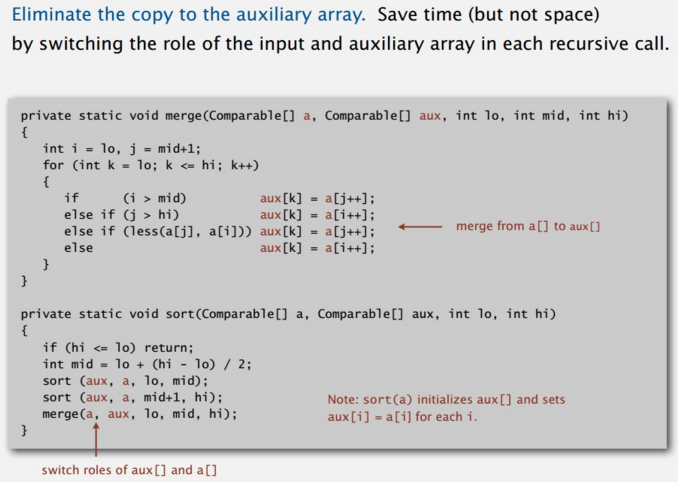适用范围

如果你对空间要求不高，且想要一个稳定的算法。那么可以使用归并排序。

快速排序

传说中最快的排序算法，听说能裸写快排，月薪可上10k...

快排平均情况下时间复杂度O(nlogn)，最糟糕情况O(n²)。O(n²)主要是因为选定的主元是极端值造成的，比如说最大值，最小值。不过这种情况一般很少出现，所以在进行快排之前我们需要对数组进行乱序，尽量避免这种情况的发生。

原理

第一步打乱数组。

然后也是分治法。归并是先分再合并。快排是先排序再分别排序两边。

排序过程核心思想是为了选出一个数，把数组分成左右两边，左边比主元小，右边比主元大。

选定第一个数作为主元。然后设定两个index指向数组首尾，比如i，j。接着从两边向中间扫描，分别用a[i]和a[j]和主元比较。若两边位置不对则交换a[i]和a[j]，比如说a[i]在扫描过程中遇到a[i]>主元，那么则停止扫描,因为我们需要左边的数小于主元,反正右边也一样等到a[j]也停下来,则交换a[i]和a[j]。

得到中间的位置之后再分别左右递归排序。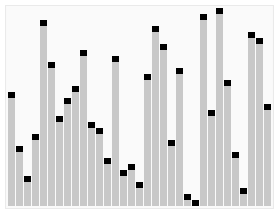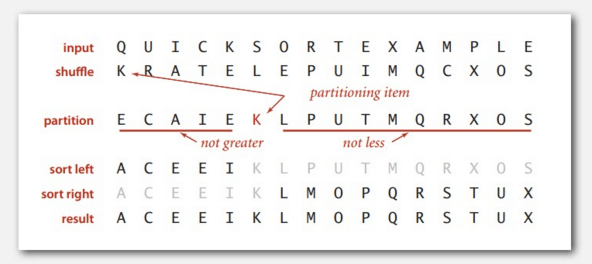实现

import com.anxpp.sort.base.Sortable;
/**
* 快速排序
* @author u
*
* 原理：选择一个基准元素，通过一趟扫描，将数据分成大于和不大于基准元素的两部分（分别在基准元素的两边），此时
*       基准元素就在未来排好后的正确位置，然后递归使用类似的方法处理这个基准元素两边的部分。
* 既然用了递归，难免在空间上的效率不高...
* 平均性能通常被认为是最好的
*/
public class quickSort extends Sortable {
public quickSort(){
super.LABLE = "快速排序";
}
/**
*
* @param a 要排序的列表
* @param low 左边位置
* @param high 右边位置
*/
private void sort(int[] a,int low,int high){
//左
int l =low;
//右
int h = high;
//基准值
int k = a[low];
//判断一趟是否完成
while(l<h){
//若顺序正确就比较下一个
while(l<h&&a[h]>=k)
h--;
if(l<h){
int temp = a[h];
a[h] = a[l];
a[l] = temp;
l++;
}
while(l<h&&a[l]<=k)
l++;
if(l<h){
int temp = a[h];
a[h] = a[l];
a[l] = temp;
h--;
}
}
if(l>low) sort(a,low,l-1);
if(h<high) sort(a,l+1,high);
}
@Override
public void sort(int[] a) {
sort(a,0,a.length-1);
}
}

优化

第一步的随机打乱数组，虽然会耗费一定时间，但却是必要的。同样的小数组的排序，快排不如插入排序。所以小数组可以直接采用插入排序。主元的选择方式可以有多种，比如随机选择主元。或者选取三个数，取中位数为主元，但是会耗费一定时间。

适用范围

虽然快速排序是不稳定的。但快速排序通常明显比其他Ο(nlogn)算法更快，因为它的内部循环很小。快速排序在对重复数据的排序时，会重复划分数据进行排序。虽然性能也还行，但这里可以进行改进，就是下面介绍的三向切分排序。

三向切分

快速排序的一种改进，使快排在有大量重复元素的数据，同样能保持高效。

原理

基本原理和快排差不多。三向切分的时候在划分数组时不是分为两组，而是分成三组。

•     小于主元
•     和主元相等
•     大于主元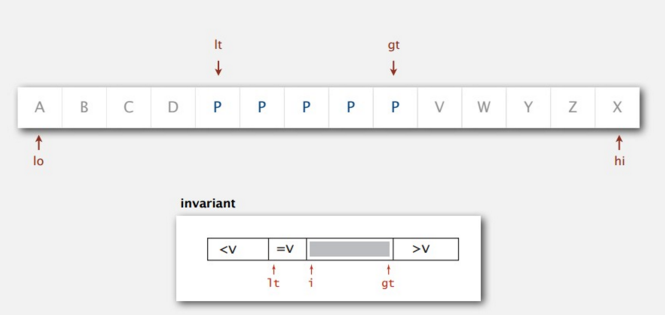实现

public class ThreeWaySort extends Sortable {
public void sort(int[] a,int l ,int h) {
if(l>=h) return;
int v = a[l];
int i=l;
int lv=l;
int gh=h;
while(i<=gh){
int cmpIndex = compare(a[i],v);
if(cmpIndex<0) exch(a,i++,lv++);
else if(cmpIndex>0) exch(a,i,gh--);
else i++;
}
sort(a,l,lv-1);
sort(a,gh+1,h);
}
@Override
void sort(int[] a) {
sort(a,0,a.length-1);
}
}

堆排序

时间复杂度O(nlogn)，堆排序主要用二叉堆实现，在讲堆排序之前我们可以要先了解下二叉堆。

二叉堆

所谓的二叉堆用一颗二叉树表示，也就是每一个节点都大于它的左右子节点。也就是说根节点是最大的。

二叉树用数组存储，可以用下标来表示节点。比如i这个节点的父节点为i/2，左儿子为2*i，右儿子为2*i+1.

堆的操作主要有两种上浮和下沉。主要对应两种情况，比如在数组末尾添加节点，此时需要上浮节点，保证二叉堆的特点。反之在替换根节点是则需要下沉操作。

原理

分为两步。

•     把数组排成二叉堆的顺序
•     调换根节点和最后一个节点的位置，然后对根节点进行下沉操作。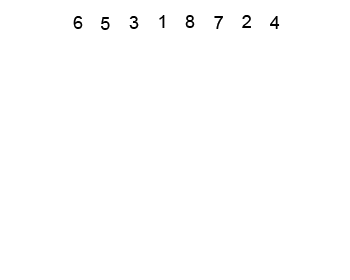适用范围

堆排序也是不稳定的。

堆排序在空间和时间上都是O(nlogn),且没有最糟情况，但在平均情况下比快排慢。

所以现在大部分应用都是用的快排，因为它的平均效率很高，几乎不会有最糟情况发生。

但如果你的应用非常非常重视性能的保证，比如一些医学上的监控之类的。

那么可以使用堆排序。还有一个堆排序的缺点，是它无法利用缓存，几乎很少和相邻元素的比较。

运行时间比较

使用下面的代码测试以上排序算法：

import java.util.Random;
import com.anxpp.sort.base.Sortable;
/**
* 测试排序算法
* @author anxpp.com
*
*/
public class TestSort {
//需要排序的数字长度为LEN
private final static int LEN = 30000;
//最大值为MAX
private final static int MAX = 99999;
public static void main(String[] args){
//初始化排序算法
Sortable[] sortables = {
new BubbleSort(),new BetterBubbleSort(),new SelectionSort(),
new InsertionSort(),new ShellSort(),new MergeSort(),
new BetterMergeSort(),new quickSort(),new ThreeWayQuickSort()};
//产生源数据
Random random = new Random();
random.setSeed(System.currentTimeMillis());
int[][] a = new int[sortables.length][LEN];
int i = 0;
while(i++ < LEN-1){
int num = random.nextInt(MAX);
int j = 0;
while(j<sortables.length)
a[j++][i] = num;
}
//排序
for(i = 0;i<sortables.length;i++){
System.out.println(sortables[i].getLABLE()+":");
//        	print(a[i]);
//        	sortTime(a[i],sortables[i]);
System.out.println(sortTime(a[i],sortables[i]));
//        	print(a[i]);
}
}
public static int sortTime(int[] a,Sortable sortable){
long start = System.currentTimeMillis();
sortable.sort(a);
return (int) (System.currentTimeMillis()-start);
}
public static void print(int[] a){
for(int i = 0;i<a.length;i++)
System.out.print(a[i] + ",");
System.out.println();
}
}

下面是本人的一次运行结果：

2348

2660

250

907

12

7

5

6

15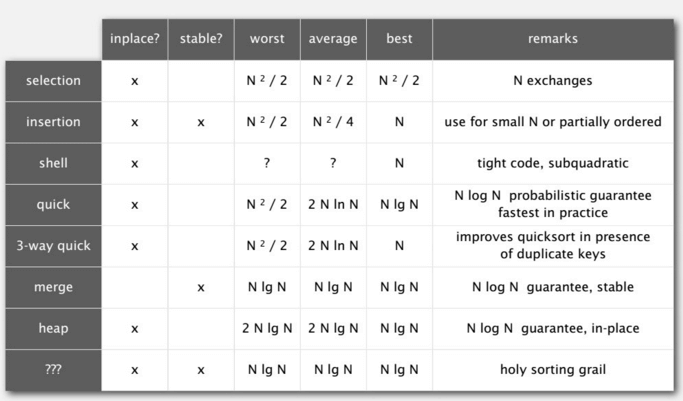推荐一个很好的网站，对各种算法进行了总结，和动画描述:sorting-algorithmsEvyn_lv

PHP常见排序算法学习

moTzxx
2017/10/27
0
0

cmazxiaoma
2017/11/12
0
0
PHP常见排序算法整理学习

u011415782
2017/10/24
0
0

2016/12/11
0
0

Vincent Ko
2018/08/15
0
0

35分钟前
6
0
JS内嵌多个页面,页面之间如何更快捷的查找相关联的页面

36分钟前
7
0
Hibernate 5 升级后 getProperties 错误

honeymoose
37分钟前
6
0
mysql-connector-java升级到8.0后保存时间到数据库出现了时差

ValSong
40分钟前
7
0
Spring中BeanFactory与FactoryBean的区别

43分钟前
5
0